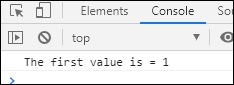# RxJS - Filtering Operator first

This operator will give the first value emitted by the source Observable

## Syntax

```first()
```

## Return value

An observable will be returned with the first value.

## Example

```import { of } from 'rxjs';
import { first } from 'rxjs/operators';

let all_nums = of(1, 6, 5, 10, 9, 20, 40);
let final_val = all_nums.pipe(first());
final_val.subscribe(x => console.log("The first value is = "+x));
```

The first() operator gives the first value from the list given.

## Output